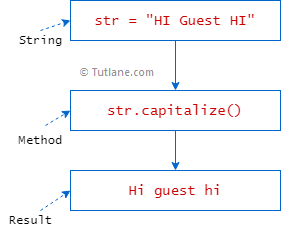# Python String Capitalize Method

In python, the string capitalize() method is useful to convert the first letter of the given string to uppercase and remaining all characters to lowercase.

Following is the pictorial representation of string `capitalize()` method functionality in python.If you observe the above diagram, we are trying to capitalize the given string “`HI Guest HI`” using the `capitalize` function in python.

## String Capitalize Method Syntax

Following is the syntax of defining a string capitalize method in python.

string.capitalize()

The python capitalize method will not accept any parameters.

## String Capitalize Method Example

Following is the example of converting the first letter of the given string to uppercase and remaining all characters to lowercase using the string `capitalize()` method in python.

msg1 = "HI Guest HI"
print(msg1.capitalize())
msg2 = "welcome to tutlane"
print(msg2.capitalize())
msg3 = "2LEARN PYTHON"
print(msg3.capitalize())

If you observe the above example, we are converting the first letter of a given string to uppercase using the `capitalize()` method.

When you execute the above python program, you will get the result as shown below.

Hi guest hi
Welcome to tutlane
2learn python

This is how you can use the string `capitalize()` method in python to convert the first letter of given strings to uppercase based on your requirements.